Courses

# Sample JEE Main Chemistry Mock Test

## 25 Questions MCQ Test | Sample JEE Main Chemistry Mock Test

Description
This mock test of Sample JEE Main Chemistry Mock Test for JEE helps you for every JEE entrance exam. This contains 25 Multiple Choice Questions for JEE Sample JEE Main Chemistry Mock Test (mcq) to study with solutions a complete question bank. The solved questions answers in this Sample JEE Main Chemistry Mock Test quiz give you a good mix of easy questions and tough questions. JEE students definitely take this Sample JEE Main Chemistry Mock Test exercise for a better result in the exam. You can find other Sample JEE Main Chemistry Mock Test extra questions, long questions & short questions for JEE on EduRev as well by searching above.
QUESTION: 1

### 1-Phenylethanol can be prepared by the reaction of benzaldehyde with:

Solution: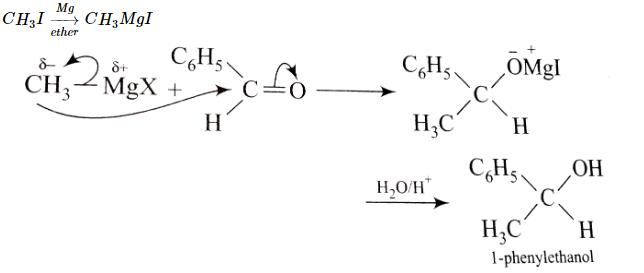QUESTION: 2

### Phenols are more acidic than alcohols because ______.

Solution:

Phenol is more acidic than cyclohexanol and acyclic alcohols because the phenoxide ion is more stable than the alkoxide ion. In an alkoxide ion, such as the one derived from cyclohexanol, the negative charge is localized at the oxygen atom.

QUESTION: 3

### Which is most ionic?

Solution:

For a compound to be ionic there must be:

• large cation
• small anion.

Lesser the number of charge, which is there in MnO.

QUESTION: 4

Electrophiles are ______.

Solution:
• An electrophile is a reagent attracted to electrons.
• Electrophiles are positively charged or neutral species having vacant orbitals that are attracted to an electron rich centre.
• It participates in a chemical reaction by accepting an electron pair in order to bond to a nucleophile.
QUESTION: 5

The effect of atoms or groups loosing electrons towards a carbon atom is called:

Solution:
• Polarity produced in a molecule due to transmission of unequal sharing/partial displacement of the bonding electron through a chain of atoms in molecules towards more electronegative atom, giving rise to a permanent dipole in a bond.
• It is present in a sigma bond, as opposed to the electromeric effect, which is present on a π-bond. it's called +I effect.
QUESTION: 6

Electronic configuration of deuterium atom is:

Solution:
• Deuterium is also known as "Heavy Hydrogen".
• A Deuterium atom consists of a proton, a neutron and an electron.
• It is just like the Hydrogen, but only with a. neutron.
• So, electronic configuration is same as that of Hydrogen: 1s¹.
QUESTION: 7

If principal quantum no. of atom is 3, then its azimuthal quantum number is:

Solution:

Principle quantum no. (n) represent shell no. while azimuthal quantum no. (l) represent sub shell no.

QUESTION: 8

Among the following the achiral amino acid is:

Solution:

It is achiral as it has two (−CH2OH)(−CH2OH) groups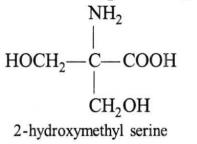QUESTION: 9

Which of the following is paramagnetic species?

Solution:

KO2 is a superoxide having anion as( O2)^(-1) and so number of valence electrons = odd and hence it is paramagnetic

QUESTION: 10

The IUPAC name for the complex
[Co(NO2) (NH3)5] Cl2 is :

Solution:

The IUPAC name of the given coordination complex according to the nomenclature rules is Pentamminenitrito-N-cobalt(III)chloride. Hence the correct option is B.

QUESTION: 11

How many EDTA (ethylenediaminetetraacetic acid) molecules are required to make an octahedral complex with a Ca2+ ion?

Solution:

An octahedral complex has 6 bonds around the central atom. One EDTA molecule complexes with one molecule of calcium cation to form octahedral complex.

QUESTION: 12

The correct IUPAC name of the structure given below is :Solution:
QUESTION: 13

Low spin complexes are generally formed by the elements of:

Solution:

This is because pairing in these (5d & 4d series) elements does not depend upon strength of ligand. it takes almost always, forming low spin complex, unlike 3-d series elements , pairing in 3d series elements depends upon strength of ligand.

QUESTION: 14

Which of the following is not correct?

Solution:

There are three sigma bonds and three pi bonds showing a hybridization type: sp3 type.

QUESTION: 15

When HCl gas is passed through a saturated solution of common salt, pure NaCl is precipitated because

Solution:
• Solubility of NaCl decreases.
• Because of the increase in Cl- ions from the HCl , common ion effect takes place and ionisation of NaCl decreases reducing its solubility.
• Hence, correct answer is (D).
QUESTION: 16

But-1-ene and cyclobutane exhibit :

Solution:

But-1-ene and cyclobutane exhibits ring chain isomerism.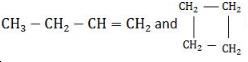QUESTION: 17

Which of the following is used in paints?

Solution:

It is a thermosetting plastic. It is obtained by condensation of ethylene glycol and phthalic acid or glycerol and phthalic acid. When its solution in a suitable solvent is evaporated, it leaves a tough but non-flexible film. It is, therefore, used in the manufacture of paints and lacquers.

QUESTION: 18

Which of the following statement is correct with respect to property of elements with an increase in atomic no. in the carbon family?

Solution:
• In carbon family, C and Si show +4 oxidation state. The remaining elements of this group, i.e., Ge, Sn and Pb, however show two oxidation states +2 and +4 due to inert pair effect which arises due to ineffective shielding of the valence s-electrons by the intervening d- or f-electrons.
• Evidently, as the number of d and f-electrons increases down the group from Ge to Pb, the inert pair effect becomes more and more prominant.
• As a result, the stability of +4 oxidation state decreases while that of the +2 oxidation state increases from Ge to Pb.
QUESTION: 19

A non-volatile electrolyte dissolved in an aqueous solution in same molal proportion as non-electrolyte produces ______.

Solution:
• The vant Hoff factor for the non electrolyte is equal to 1 whereas that of non volatile electrolyte is greater than 1.
• Hence all colligative properties will be increased.
QUESTION: 20

Which of the following alkali metals has the highest tendency for the half cell reaction?
M(g)   → M(g)+  + e-

Solution:

As the given reaction is oxidation reaction and lithium has highest oxidation potential, so it has highest tendency for this half reaction.

*Answer can only contain numeric values
QUESTION: 21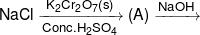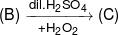Determine total number of atoms in per unit formula of (A), (B) & (C).

Solution:

(A) = CrO2Cl2
(B) = Na2CrO4
(C) = CrO5

*Answer can only contain numeric values
QUESTION: 22

4 mole of a mixture of Mohr's salt and Fe2(SO4)3 requires 500 mL of 1 M K2Cr2O7 for complete oxidation in acidic medium. The mole % of the Mohr's salt in the mixture is :-

Solution:

FeSO4 (NH4)2 SO4 . 6H2O + Fe2 (SO4)3
Mohr salt
x mol               (4–x) mol
∵ (Eq) K2Cr2O7 = (Eq) Mohr solt
(Cr+6 → Cr+3)    (Fe+2 → Fe+3)
N × V(L)                        = mol × n factor
1 × 6 × 0.5                   =  x × 1
x = 3 mol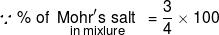= 75

*Answer can only contain numeric values
QUESTION: 23

The half-life of decomposition of gaseous CH3CHO at initial pressure of 364 mm and 182 mm of Hg were 440 sec and 880 sec respectively. The order of the reaction is :-

Solution: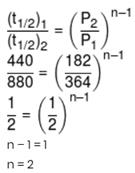*Answer can only contain numeric values
QUESTION: 24

If rate of formation of SO3 is 0.8 g.lit–1.sec–1 then calculate the rate of disappearance of O2 in g.lit–1.sec–1 for the reaction 2SO2 + O2 → 2SO3

Solution: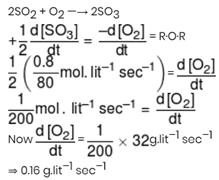*Answer can only contain numeric values
QUESTION: 25

The total number of possible isomers with molecular formula C6H12 that contain a cyclobutane ring.

Solution: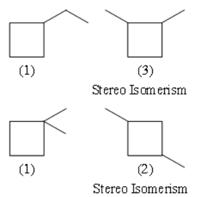Track your progress, build streaks, highlight & save important lessons and more!

### Similar Content### Related tests